## Resistance

What is resistance?

A third factor that plays a role in an electrical circuit is resistance. All material impedes the flow of electrical current to some extent. The amount of resistance depends upon composition, length, cross-section and temperature of the resistive material. The resistance of a conductor increases with an increase of length or a decrease of cross section. Resistance is designated by the symbol “R”. The unit of measurement for resistance is ohms (Ω).

Resistor Symbols

Resistance is usually indicated symbolically on an electrical drawing by one of two ways. An unfilled rectangle is commonly used. A zigzag line may also be used.

Resistance can be in the form of various components. A resistor may be placed in the circuit, or the circuit might contain other devices that have resistance.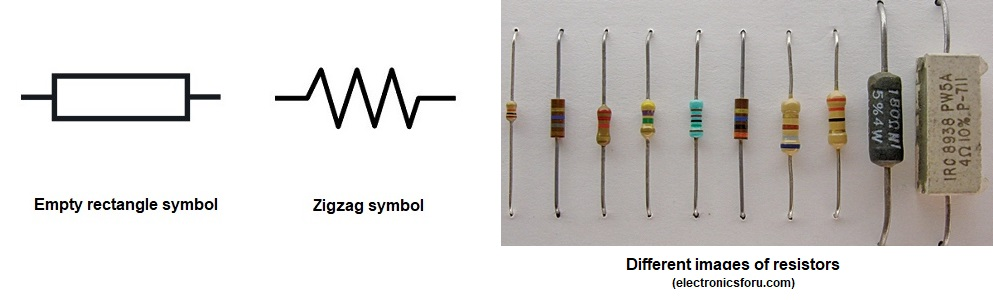Other Resistor Images

Other resistor images used to limit or reduce the current  in a circuit to accomplish a purpose. Resistors are also used to modify the functions of different parts of a circuit or a device. Most resistors are color coded if the value of resistance is not stated, which is in Ohms.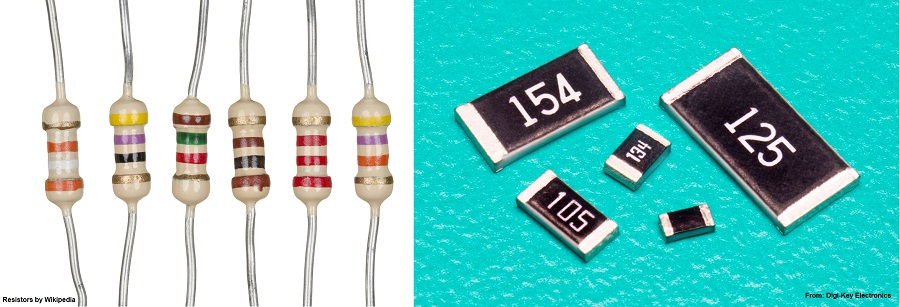You notice that some resistors, if the resistance value is not indicated numerically, are color coded. These indicate the resistance value and other relevant information you need to know to be able to effectively use it in the right place and where it is needed.  Below are the colors, their numeric values and their meanings.

Resistor Color Coding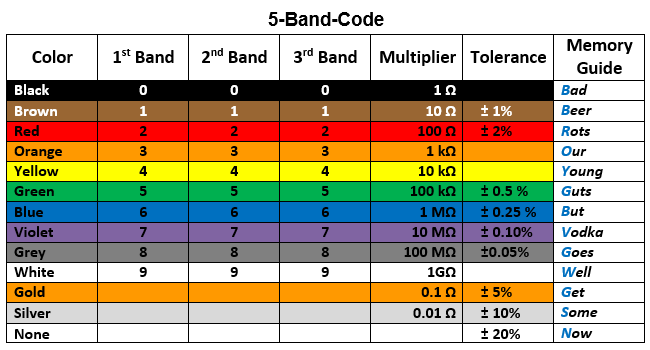Resistor Color Coding

Here is an example of a color-coded resistor along with the interpretation of the codes.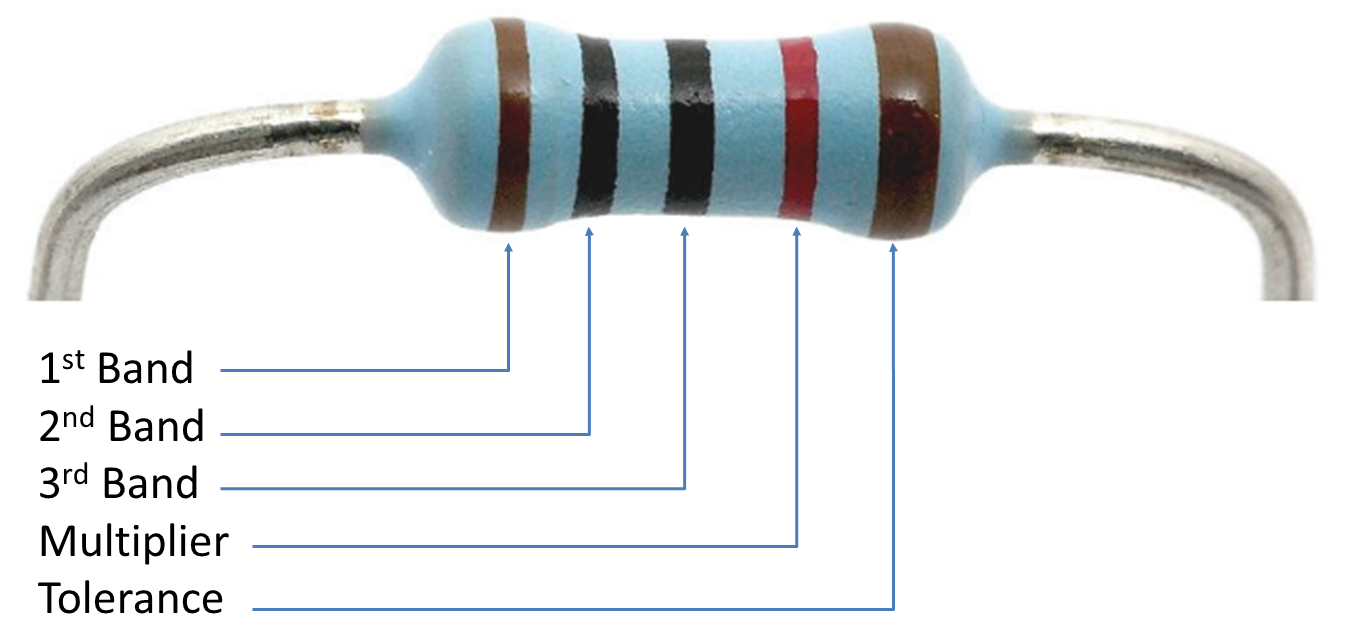Color Coding Interpretations

Here is an example of a color-coded resistor along with the interpretation of the codes.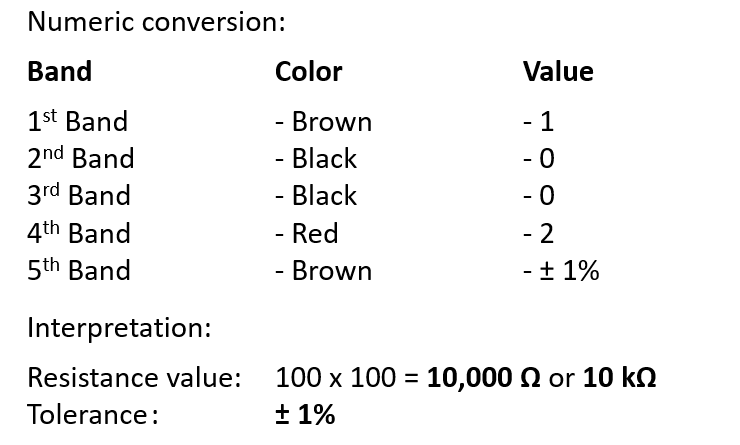Units of Measurement

The table below explains the unit used in measuring resistance where prefixes were used when dealing with very small or large values of resistance.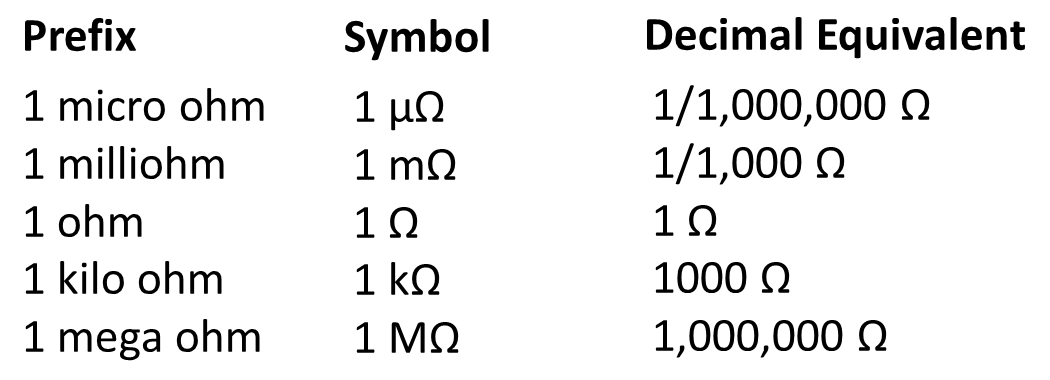Posted in